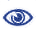# Prague Stringology Conference 2020

Simple KMP Pattern-Matching on Indeterminate Strings

 Abstract: In this paper we describe a simple, fast, space-efficient approach to finding all matches of an indeterminate pattern p = p[1..m] in an indeterminate string x = x[1..n], where both p and x are defined on a ❝small❞ ordered alphabet Σ — say, σ = |Σ| ≤ 9. A preprocessing phase replaces Σ by an integer alphabet ΣI of size σI = σ that (reversibly, in time linear in string length) maps both x and p into equivalent regular strings y and q, respectively, on ΣI, whose maximum (indeterminate) letter can be expressed in a 32-bit word (for σ ≤ 4, thus for DNA sequences, an 8-bit representation suffices). We then describe an efficient version KMP_Indet of the venerable Knuth-Morris-Pratt algorithm to find all occurrences of q in y (that is, of p in x), but, whenever necessary, using the prefix array, rather than the border array, to control shifts of the transformed pattern q along the transformed string y. Although requiring O(m2n) time in the theoretical worst case, in cases of practical interest KMP_Indet executes in O(n) time. A noteworthy feature is the very small additional space requirement: Θ(m) words in all cases. We conjecture that a similar approach may yield practical and efficient indeterminate equivalents to other well-known pattern-matching algorithms, especially Boyer-Moore and its variants.

 Download paper:PostScript PDF BibTeX reference
 Download presentation: